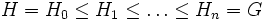Subabnormal subgroup

This article defines a subgroup property: a property that can be evaluated to true/false given a group and a subgroup thereof, invariant under subgroup equivalence. View a complete list of subgroup properties[SHOW MORE]

Definition

Definition with symbols

A subgroup$H$ of a group$G$ is termed subabnormal if there exists an ascending chain:$H = H_0 \le H_1 \le \ldots \le H_n = G$

such that each$H_i$ is an abnormal subgroup of$G$.

Metaproperties

Transitivity

This subgroup property is transitive: a subgroup with this property in a subgroup with this property, also has this property in the whole group.
ABOUT THIS PROPERTY: View variations of this property that are transitive | View variations of this property that are not transitive
ABOUT TRANSITIVITY: View a complete list of transitive subgroup properties|View a complete list of facts related to transitivity of subgroup properties |Read a survey article on proving transitivity